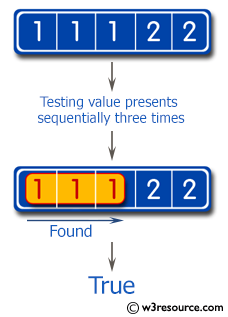﻿ Swift Basic Programming Exercise: Test whether a value presents sequentially three times in an array of integers or not - w3resource# Swift Basic Programming Exercise: Test whether a value presents sequentially three times in an array of integers or not

## Swift Basic Programming: Exercise-28 with Solution

Write a Swift program to test whether a value presents sequentially three times in an array of integers or not.

Pictorial Presentation:Sample Solution:

Swift Code:

``````func test_Triples(_ input: [Int]) -> Bool {
for (index, number) in input.enumerated() {
let thirdIndex = index + 2
let secondIndex = index + 1

if secondIndex < input.endIndex && number == input[secondIndex] && number == input[thirdIndex] {
return true
}
}
return false
}
print(test_Triples([1, 1, 1, 2, 2]))
print(test_Triples([1, 1, 1, 2, 2, 2, 2]))
print(test_Triples([1, 1, 3, 3, 1]))
```
```

Sample Output:

```true
true
false
```

Swift Programming Code Editor:

Improve this sample solution and post your code through Disqus

What is the difficulty level of this exercise?

﻿